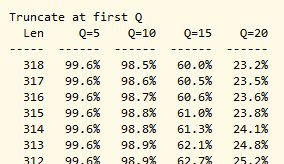fastq_stats command

Report statistics on reads in a FASTQ file. Useful for choosing FASTQ filter parameters.

Output is written to a file specified by the -log filename command-line option.

FASTQ format options are supported, these must be specified if the Q scores are specified using non-default ASCII characters.

Example

Reported statistics
The log file format is subject to change. It is meant to be human-readable rather than parsed by a script. It would be nice to have an option to write the information to a format like tabbed text that can easily be parsed or imported into a program such as Excel that can make charts. Hopefully I will add this feature in the near future; please let me know if you need this.

Length distribution
This section reports the read length distribution. Columns are: L=read length, N=number of reads, Pct=fraction of reads with this length, AccPct=fraction of reads that are greater than or equal to this length.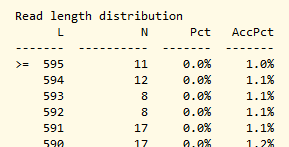Q score distribution
This section reports the number of bases found for each Q score. Columns are: ASCII=symbol, Q=integer Phred score, N=number of bases, Pct=number of bases with this Q score, AccPct=number of bases with >= this Q score.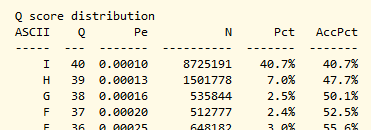Length vs. quality distribution
This section shows the fraction of records and average expected number of errors obtained by truncating at each possible position in the read. Columns are: L=position in read, PctRecs=fraction of reads with at least this length, AvgQ=average Q score over all reads obtained by truncating at this position, P(AvgQ)=error probability corresponding to AvgQ, AvgP=average error probability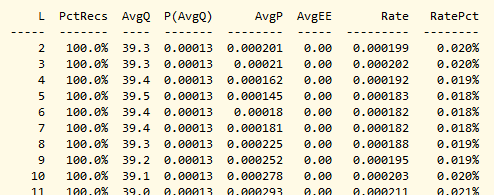Expected error truncation summary
This section summarizes the effect of some common choices of max. expected errors trunction (fastq_filter command with -fastq_maxee and -fastq_trunclen options). L=truncation length, columns 1.0, 0.5, 0.25 and 0.1 give the number of reads and the fraction of reads respectively that would pass a filter with the options given (-fastq_trunclen=L and -fastq_maxee=1.0, 0.5, 0.25 or 0.1).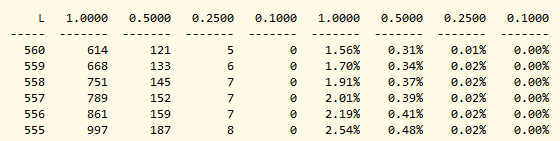Minimum Q truncation summary
This section summarizes the effect of length truncation with some common choices of minimum Q (fastq_filter command with fastq_truncqual and fastq_trunclen options). Len is the truncation length (fastq_trunclen option), the other columns give the fraction of reads that pass that filter.